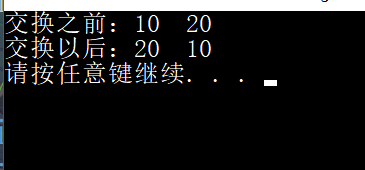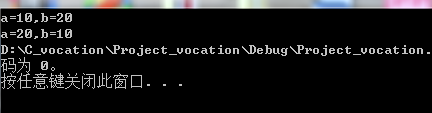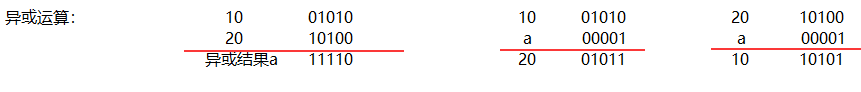• 交换整型变量的值，最简单的就是创建临时变量，作为桥梁进行交换。 #include <stdio.h> int main() { //创建两个整数，并打印当前数值 int a = 10; int b = 20; printf("交换之前：%d %d\n", a, b); /...
给定两个整形变量的值，将两个值的内容进行交换。
交换整型变量的值，最简单的就是创建临时变量，作为桥梁进行交换。
#include <stdio.h>

int main()
{
//创建两个整数，并打印当前数值
int a = 10;
int b = 20;
printf("交换之前：%d  %d\n", a, b);
//创建临时变量，当做a和b交换的桥梁。交换之后打印
int x = a;
a = b;
b = x;
printf("交换以后：%d  %d\n", a, b);
system("pause");
return 0;
}
实验结果：还有一种方法，不用临时变量的话进行交换，那就是 使用异或运算符操作。
#include<stdio.h>

int main()
{
//创建两个整型变量，打印
int a = 10;		//10=0 1010
int b = 20;		//20=1 0100
printf("交换之前：%d  %d\n", a, b);
//进行异或操作，并打印结果,
a = a ^ b;		//0 1010^1 0100=1 1110
b = a ^ b;		//1 1110^1 0100=0 1010=10
a = b ^ a;		//0 1010^1 1110=1 0101=20
//由此实现不创建临时变量就可以交换两个整型变量的值
printf("交换之后：%d  %d\n", a, b);
system("pause");
return 0;
}
实验结果：展开全文• 写一个函数交换整型变量内容 本网所有内容文字、图片和音视频资料，版权均属个人所有，仅供个人呢学习参考，任何媒体、网站或个人未经本网协议授权不得转载、链接、转贴或以其他方式复制发布/发表。 #include<...
写一个函数交换整型变量内容
本网所有内容文字、图片和音视频资料，版权均属个人所有，仅供个人呢学习参考，任何媒体、网站或个人未经本网协议授权不得转载、链接、转贴或以其他方式复制发布/发表。
#include<stdio.h>
void swap(int* pa,int* pb)//存放a,b的地址
{
int c = 0;
c = *pa;
*pa = *pb;
*pb = c;
}
int main()
{
int a = 10;
int b = 20;
printf("a=%d,b=%d\n", a, b);
swap(&a, &b);//交换地址，所以结果交换成功
printf("a=%d,b=%d", a, b);
return 0;
}展开全文c语言
• 交换，却不给空间暂存，我们只有“自力更生”，在中间信息存到这两个变量仅有的空间里了。 这样的思路下来，很自然的就想到了位操作。 a = a^b; b = a^b; a = a^b; 其实用加法也可以，不过会溢出...

要交换，却不给空间暂存，我们只有“自力更生”，在中间信息存到这两个变量仅有的空间里了。

这样的思路下来，很自然的就想到了位操作。

a = a^b;
b = a^b;
a = a^b;

其实用加法也可以，不过会溢出：
a = a+b;
b = a-b;
a = a-b;


展开全文• 给两个整型变量赋值，然后交换两个变量的值，最后输出交换后的变量值。
• 交换两个整型变量的值，有以下三种方法：创建临时变量；求和做差；按位异或。1.创建临时变量：引入一个变量tmp存放其中一个待交换变量a，再对待交换变量赋值即可。#include &lt;stdio.h&gt; int main() { ...
交换两个整型变量的值
有以下三种方法：1.创建临时变量；2.求和做差；3.按位异或。
1.  创建临时变量：引入一个变量tmp存放其中一个待交换变量a，再对待交换变量赋值即可。
#include <stdio.h>
int main()
{
int a = 10;
int b = 20;
int tmp = 0;

scanf("%d%d", &a, &b);
printf("a = %d  b = %d\n", a, b);

tmp = a;
a = b;
b = tmp;

printf("a = %d  b = %d\n", a, b);
system("pause");
return 0;
}

2.   在不引入变量的前提下，交换两个数的内容。其思路是，将这两个数求和然后赋给其中一个变量a，变量a减去变量b就能得到原来的a的值。
但是，这个方法存在一定的局限性，如果参与交换的两个数比较大，那么a+b的值大小 会超过整型数据类型分配的内存空间，使值溢出，结果出现错误。
#include <stdio.h>
int main()
{
int a = 10;
int b = 20;

scanf("%d%d", &a, &b);
printf("a = %d, b = %d\n", a, b);

a = a + b;         //a的值被重新赋值，现在a=30,    b=20
b = a - b;         //a-b 的值是原来a的值   将这个结果赋给b,则b已经完成了交换,b的值发生了改变    即b中现在是原来a的值
a = a - b;         //a-b 的值是原来b的值   将这个结果赋给啊，完成整型变量的交换

printf("a = %d,b = %d\n", a, b);
system("pause");
return 0;

}

3.   按照（二进制）位异或 的方法交换数据。在计算机中，所有的数据都是以二进制0,1形式存储的；比如10 在计算机中的存储序列为1010，  20在计算中的存储序列为 10100。 按位异或的规则是：不同为1，相同为0，即对应二进制位上 是1和0时，结果为1，否则为0；所以，异或的作用就是产生了一段序列。当参与异或运算的数遇到这一段序列时，会翻译出另外一个数。可以类比生物学中的tRNA参与翻译的过程来帮助理解。
因此，代码可以写成：
#include <stdio.h>
int main()
{
int a = 0;
int b = 0;
scanf("%d%d",&a,&b);
printf("a = %d    b = %d", a, b);   // /假设输入a=10, b=20;         a 二进制序列：01010
//                             b 二进制序列：10100
a = a ^ b;                          //                             a =          11110
b = a ^ b;
a = a ^ b;

printf("\na = %d    b = %d\n", a, b);
return 0;
}



展开全文c 语言
• #include<stdio.h>#include<conio.h>...//定义一个函数作用是交换两个整型变量的数值 int x,y;//定义整型变量x，y int *a1,*a2;//定义整型指针a1，a2 printf("任意输入两个数值："); s...
• 给定两个整型变量的值，将两个值得内容进行交换 创建一个临时变量k，来实现变量a和b的交换 #include &lt;stdio.h&gt; #include &lt;stdlib.h&gt; int main() { int a = 3; //定义一个整型变量...
• #include<stdio.h> int main() { ...//定义了两个整型变量 scanf("%d%d",&a,&b);//用输入函数输入两个整型变量 int *p,*q;//定义两个指针变量p=&a; q=&b;//把指针p,q分别指向a,...
• 交换两个整型变量的方式，我们一般都会借助一个临时变量来交换。在这里，不用临时变量，直接交换连个整型变量的值。方法一：int a=3,int b=8; //交换a和b的值 n=n+m; m=n-m; n=n-m; //这种方法比较容易造成溢出，...
• 在一次面试中，做了这么一道题"交换两个整型变量的值"，当时看到这个题目之后，会心一笑，这也太简单了--直接使用中间变量交换不就可以了吗？但是，面试官却说不需要返回值，在调用的地方，再次输出变量的值，我就不...
• c语言定义一个函数，实现交换两个变量的值，需要传递变量的地址（指针），这样能够保证 swap() 函数交换的是两个指针指向的整型数据。如果只是传递变量的话，例如 try_change()， 交换的是在这个函数体内的变量值，...c语言 指针
• // 有以下两个变量 a，b，需求对其中进行交换值，使 a = 2，b = 1 int a = 1; int b = 2; 通过第三个变量交换 int c = a; // c = 1 a = b; // a = 2 b = c; // b = 1 不通过第三个变量交换 通过表达式 a = a + ...算法
• 交换两个整型变量，不使用第三个变量，实现两个变量的交换（这是公开课上听到的，觉得开阔了思路，所以分享给大家看一看） 下面以a=3,b=5,进行举例第一种是转换的问题：目标：实现a和b的交换 首先1.a=a+b;此时，b是...算法 经验分享 程序人生
• 在编程中，经常容易遇到交换两个变量的情况，比如实现“找出两个整型变量m,n的最小公倍数和最大公约数”的算法时，一般的算法开始找出两个数中最大的数然后进行交换给m,最小的数交换给n,然后实现运算。在交换的过程...算法 编程
• 给定两个整型变量i，j，交换两者的值。 方式一：定义一个临时变量tmp,然后根据临时变量交换两个变量的值。 你以为这就对了吗？细想一下，如果i，j是负数呢？ 方式二：不设立临时变量，用加法操作交换两个变量的...
• #define _CRT_SECURE_NO_WARNINGS 1 #include<stdio.h> #include<string.h> void Swap(int* pa, int* pb) { int temp = 0; temp = *pb;... printf("交换前a=%d b=%d\n", a, b); Swap(&a,&c++
• 第一种方法，对两个数进行交换，我们首先想到的就是创建临时变量，实现两变量交换 例如： int a=10,b=20,t; t=a; a=b; b=t; 第二种,不创建临时变量,利用数学的思想对两个数进行交换。 如下： int a=10，b=20; a=...
• 最后将临时变量temp赋值给a即可（完成交换） 下面是例子： 输出结果为： 2.初学者 1.我们可以把两个变量（假设为a,b）的和假设成第三方变量（sum）。 2.当我们要进行交换的时候 变量a = sum-a;(这样不就完成了将b...JAVA 方法
• 交换两个整型变量(不借助临时变量)这是多年前我做Java时面试碰到的题目，其实不难。特意写下这篇博客，是因为我发现有不止一种方法可以实现，原谅我无聊的双手。图1先简单说说普通的方法吧，如上图：int i = 5;int p...算法
• 突破口：用其中一个变量暂存两者的和。a=a+b; b=a-b;//此时b已经是a a=a-b;//相当于(a+b)-a=b 转载于:https://blog.51cto.com/happyyoung/1762744...
• //将两个整型变量的值进行交换 #include <stdio.h> int main() { int a, b, c; a = 1; b = 2; printf("a=%d, b=%d\n", a, b); c = a; a = b; b = c; printf("a=%d，b=%d\n", a, b); return 0; } ......